# Resistance

it is the property by virtue of which a conductor oppose the low of charges through it.

SI unit of resistance is Ohm. 1 ohm is the resistance offered by a conductor if a current of 1 ampere flows through it on applying a potential difference of 1 volt across its ends.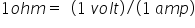Factor affecting the resistance :-

1. Length – the resistance R of a conductor is directly proportional to its length. i.e. R α I

2. Area of cross – section : the resistance R of a conductor is inversely proportional to its area of cross section: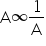Combining the above factors, we get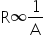Or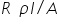Where ρ  is constant of proportionality called resistivity or specific resistance of the material of the conductor.

Specific resistance:- in the equation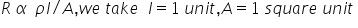Then R= ρ thus, the specific resistance of a material may be defined as the resistance of a conductor of that material having unit length and unit area of cross- section.

Or

It is the resistance offered by the unit cube of the material of a conductor.

SI unit of resistivity is ohm-m.

Current density:- the amount of charge flowing per second per unit area normal to the flow of charge. It is a vector quantity having the same direction as that of motion of positive charge.

For normal flow of charge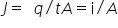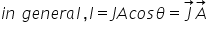SI unit is mho or Siemens or ohm-1.

Conductivity :- the reciprocal of the resistivity of a material is called conductivity and is denoted by σ.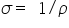SI unit is mho m-1 or Siemens m-1 or ohm-1 m-1.

Vector form of ohm’s law:- if E is the magnitude of electric field in a conductor of length I, then the Potential difference across its ends is

V = EI

Also, from ohm’s law, we can write,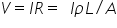E= Jρ

As the direction of current density j is same as that of electric field E, we can write the above eqn as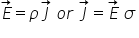The above equation is the vector form of ohm’s law. It is equivalent to scalar from, V = RI.

Related Keywords